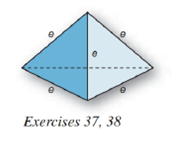Chapter 9.2, Problem 38EElementary Geometry For College St...

7th Edition
Alexander + 2 others
ISBN: 9781337614085

Solutions

Chapter
SectionElementary Geometry For College St...

7th Edition
Alexander + 2 others
ISBN: 9781337614085
Textbook Problem

Each edge of a regular tetrahedron (see Exercise 37) has length e .a) Show that the altitude of the tetrahedron measures h = 2 3 e .b) Show that the volume of the tetrahedron is V = 2 12 e 3 .c) Find the volume of the tetrahedron if each side measures e = 4 i n .To determine

(a)

To show:

The altitude of the tetrahedron measures h=23e.

Explanation

Given:

Each edge of a regular tetrahedron has length e.as below,

Properties Used:

The lateral area L of a regular pyramid with slant height of length l and perimeter P of the base is given by

L=12lP.

In a regular pyramid, the lengths of the apothem a of the base, the altitude h, and the slant height l satisfy the Pythagorean Theorem; that is, l2=a2+h2.

According to the Pythagorean theorem, in a right-angled triangle hypotenuse2=base2+perpendicular2.

Area of a right-angled triangle is 12×base×height.

Approach Used:

i) Using the property l2=a2+h2, find the height or altitude h by substituting the values of l and a.

ii) Substitute the values of base and height h in equation 12×base×height to get the area of each lateral face since it is a triangular pyramid.

iii) Compare the given value with the value from step (ii).

Calculation:

From the given figure

the sides of the square base and lateral edge all are e.

Considering the right-angled triangle with triangle base

the sides of the triangular base measure e each and the angle at the center for each base is =360°3=120° which is bisected by the perpendicular so the hypotenuses in the right-angled triangle is OA¯=side2sin60°

To determine

(b)

To show:

The volume of the tetrahedron is V=212e3.

To determine

(c)

To find:

The volume of the tetrahedron.

Still sussing out bartleby?

Check out a sample textbook solution.

See a sample solution

The Solution to Your Study Problems

Bartleby provides explanations to thousands of textbook problems written by our experts, many with advanced degrees!

Get Started

53. Demand Graph the first-quadrant portion of the demand function

Mathematical Applications for the Management, Life, and Social Sciences

In Exercises 49-62, find the indicated limit, if it exists. 56. limx2x+2x2

Applied Calculus for the Managerial, Life, and Social Sciences: A Brief Approach

Given the Taylor Series , a Taylor series for ex/2 is:

Study Guide for Stewart's Single Variable Calculus: Early Transcendentals, 8th